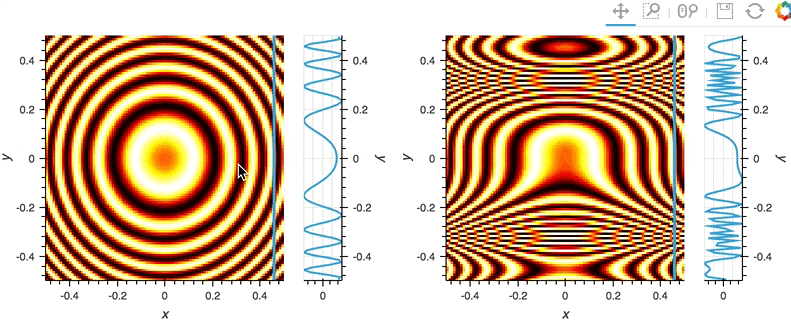# PointerX#

## Title: Linked pointer cross-section example#

Description: A linked streams example demonstrating how to use PointerXY stream linked across two plots.

Dependencies: Bokeh

Backends: Bokeh

```import numpy as np
import holoviews as hv
from holoviews import opts
hv.extension('bokeh')
``````opts.defaults(opts.Curve(width=100))

# Create two images
x,y = np.meshgrid(np.linspace(-5,5,101), np.linspace(5,-5,101))
img1 = hv.Image(np.sin(x**2+y**2))
img2 = hv.Image(np.sin(x**2+y**3))

# Declare PointerX and dynamic VLine
posx = hv.streams.PointerX(x=0)
vline = hv.DynamicMap(lambda x: hv.VLine(x or -100), streams=[posx])

# Declare cross-sections at PointerX location
# Using apply syntax
crosssection1 = img1.apply.sample(x=posx.param.x)
# By constructing a DynamicMap
crosssection2 = hv.DynamicMap(lambda x: img2.sample(x=x if x else 0), streams=[posx])

# Combine images, vline and cross-sections
((img1 * vline) << crosssection1) + ((img2 * vline) << crosssection2)
```This web page was generated from a Jupyter notebook and not all interactivity will work on this website. Right click to download and run locally for full Python-backed interactivity.